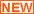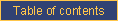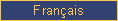homefeaturesDOCUMENTATIONpricingcontact ustechnical documentationCATI documentationcookbookrobotQuestionnaire design

Computed questions

BLANK questions

If the keyword "BLANK" is present on the question name instruction, then

• the question does not display on screen;
• the value of the first answer category is assigned to the question; and,
• eventually, the flow follows the simple skip associated with the first answer category.

A BLANK question can be associated with a display condition to attribute a value to the question conditional on previous answers or to perform complex skips.

Complex skips

A simple skip associated with the first answer category of BLANK questions is honoured. Therefore, BLANK questions can be used to implement complex skips via the display condition, i.e., the complex skip logic is placed in the display condition and the destination of the skip is associated with the first category of the question.

Complex skips can also be implemented via CALCUL questions (see the next paragraph). In this case, a calculation is made, returning a value into the same question (for example; this is not a necessity, as long as there is a value in the CALCUL question to use for skips), and response categories are associated with simple skips. For example, using the contexte system variable, the following code checks whether the current time is before or after noon and redirects the questionnaire flow accordingly:

REDIRECT CALCUL
% Text
REDIRECT = ( \$contexte{heure} < 120000 ) ? 1 : 2
% Categories
*1*AM
*2*PM
% Simple skips
1 = ~Q_AM
2 = ~Q_PM
% Display condition
% Open parts
! ===================================================<

CALCUL questions

If the keyword "CALCUL" is present on the question name instruction, CallWeb expects the question text to use the following syntax:

output_variable = perl_expression

This returns the result of the evaluation of "perl_expression" in "output_variable". The "perl_expression" must follow Perl syntax and question names must be prefixed with "\$". Please refer to the questionnaire driving Circum Network Inc.'s EchantiCalc script for several examples of use of this feature.

Some special functions can be used in a CALCUL question to perform survey-specific actions. Among them are:

 add_call_cetappel used to add a fake entry to the case call history to account for a completion over the open Web. See the CallWeb cookbook for a complete description of its use. add_to_date used to add or subtract time from a date-time value. See the CallWeb cookbook for a complete description of its use.calc_graph used as calc_graph(field => Q1), it produces a chart of the Q1 data and inserts it where the function is called. See the related recipe. combine_into_multiple used as Q5 = combine_into_multiple(\$Q1, \$Q2, ...), this function combines the answers to different (single or multiple) close-ended questions into a multiple selection value. The value returned does not contain duplicate codes.cover (or obscurcir) used as ASTRING = cover("string"), it returns an encoded version of the string that can be decoded with the uncover function. This function uses a simple encoding algorithm that returns printable ASCII characters. email used as AWHEN_EMAIL_SENT=email("blabla\@domain.com",\$AEMAIL_ADDRESS,"EMAIL_ENGLISH"), this function sends an e-mail to AEMAIL_ADDRESS based on the text in EMAIL_ENGLISH and puts the date and time of the action in AWHEN_EMAIL_SENT. Additional arguments are, in order: the width in characters of the text-only portion of the message (65 by default), the e-mail address where a cc is sent, andthe e-mail address where a bcc is sent. except_codes used as Q1B = except_codes(\$Q1,1,2,3), this function returns a multiresponse answer from which the listed codes (here, 1,2, and 3) are excluded. ferme_le_navigateur used as ""=ferme_le_navigateur(), this function sends the JavaScript code required to close the browser window. indexposition used as Q2=indexposition(number,number,number,...), it returns the position of the largest Nth number where N is the first number supplied. The largest number is in position 1. Thus, indexposition(2,1,3,5,5,7,9) returns 5 because 7 is the second largest value and it is in fifth position. last_access used as Q2=last_access("argument"), it returns information on the last access to the case. Arguments: "date" returns the 8-digit date (YYYYMMDD); "time" returns the 6-digit time (HHMMSS); "datetime" return the 14-digit date time value (YYYYMMDDHHMMSS). max used as Qx=max(number,number,number,...), it returns the largest number. min used as Qx=min(number,number,number,...), it returns the smallest number. n_calls used as AQ=n_calls(), it returns the number of calls in the case call history; used as AQ=n_calls("Code1","Code2"), it returns the number of calls with dispositions Code1 or Code2 in the case call history; used as AQ=n_calls("Code*","Nocode"), it returns the number of calls with dispositions starting with Code or dispositions Nocode in the case call history. There can be multiple arguments of this type. n_records used as Q2=n_records("CONDITION","PROJECT"), it returns the number of cases in the project data base which correspond to the condition (expressed in MySQL syntax, without the WHERE keyword). If the project name is absent, the current project is used. n_selections used as Q2=n_selections(\$Q1,1,2,3,4), it returns the number of responses in Q1 among the list of valid responses given thereafter. n_such used as NSELECT1=n_such(1,4,9,"Q1-Q10"), it returns the number of responses equal to 1, 4 or 9 among the responses to questions Q1 to Q10. positions used as POS = positions(number,number,number,...), it returns the positions of the first number in the list made up of the numbers following the first number; the positions are delimited by the multiple-answer delimiter. Thus, positions(5,1,3,5,5,7,9) returns 3µ4. pull_value used as AQX = pull_value(KEYWORD,QUESTION,PROJECT,WHERE,LIMIT), it extracts the values found in the QUESTION question of PROJECT project in cases of that project which fit the WHERE clause (phrased as a MySQL WHERE statement). The function returns a delimited list of values delimited by mu characters. KEYWORD can be "ALL" or "UNIQUE" to return all values, even duplicates or unique values. The values are returned in no particular order. Example: AQX = pull_value("ALL","myquestion","myproject","RESERVED <> 1") places a list of values from "myquestion" in "myproject" where RESERVED is different from 1 in AQX in the current project. push_value used as AQX = push_value(VALUE,QUESTION,PROJECT,WHERE,LIMIT), it places VALUE in the QUESTION field of project PROJECT in the one case of that project which corresponds to the WHERE condition (expressed in MySQL syntax). The function returns the _telkey of the case that was affected (into AQX in the example). If something goes wrong, the function returns an empty string rather than a valid _telkey value. If more than one case in the PROJECT correspond to the WHERE condition, the function also returns an empty string... unless LIMIT is the number 1 in which case the function randomly selects one of the _telkeys that correspond to the WHERE condition. Example: AQX = push_value("1","RESERVED","myproject","RESERVED <> 1",1). This will place a 1 in the RESERVED question of myproject in one case selected randomly among the cases where RESERVED does not already equal 1 and return the affected _telkey into AQX. put_values_in_case used as AQX = put_values_in_case(key=>value, key=>value, ...), it places values in fields of a given record in a given project. The complete syntax and utilization are described in the CallWeb cookbook. random_integer used as AQX = random_integer(n), it returns a random number between 1 and "n". "n" can be a fixed value like 10 or a value stored in a CallWeb field such as \$AQ1. random_integer2 used as QX = random_integer2(n,"QX"), it returns a random number between 1 and "n" into QX. "n" can be a fixed value like 10 or a value stored in a CallWeb field such as \$AQ1. The selection of the random integer is made among possible integers that have been least often selected in QX as of when the function is called. For example, looking for a random integer between 1 and 3, if 1 has already been chosed twice and 2 and 3 have never been chosen, either 2 or 3 will be returned, randomly. This ensures a flat distribution of all integers between 1 and n over time. To implement a distribution other than flat, it is possible to request a random integer between 1 and 100 and to perform different actions according to shares of the 100 integers (e.g., skip the next question if the random number is less than 51). random_subset used as COMBO = random_subset(\$MULTIQ,n), it returns a random selection of "n" answers among the answers stored in the multiple-response question "MULTIQ". The complete syntax and utilization are described in the CallWeb cookbook. recode used as Q4RECODE = recode("Q4","Q4RECODE","1,2,3=1","4-6=2","ELSE=3"), it returns the recoded value of Q4 (a close-ended question) into Q4RECODE using the rules which follow the second argument of the function. (Still using the same example) If Q4RECODE has a maximum number of answers greater than 1 (multi-response question), recode returns as many recoded categories as apply; otherwise, Q4RECODE contains the first recoded category intercepted by the rules. For example, if Q4 contains answers 1, 2 and 5, the recode returns 1 and 2 into Q4RECODE if Q4RECODE is a multiple-answer question and only 1 if it is a single-answer question. Rules following an "ELSE" rule are never tested. record_wav used as AFILENAME = record_wav("start") or PROCESSES = record_wav("stop"), this function starts or stops a recording of the feed received by the computer microphone. This is used in face-to-face interviewing. More details in the cookbook. residual used as Q3C=residual(\$Q2,\$Q3A,\$Q3B,...), it returns the responses in Q2 stripped of the responses in Q3A and Q3B (there can be any number of additional arguments); this was developed to identify the third selection in Q2 (a question allowing three selections) once the top selection in Q3A and the secondary selection in Q3B were excluded. select_random_combination used as COMBO = select_random_combination(among,number,exclusive,other_combination,other_combination,...), it returns a combination of "number" integers between 1 and "among". The complete syntax and utilization are described in the CallWeb cookbook. shuffle_code_labels used as AQy = shuffle_code_labels("Qx","[EN], [FR], ","[EN] and[FR] et"), it returns a list of the text of all answer categories in Qx, in random order, separated by the second argument; the third argument supplies the link between the second last and the last categories (typically "and", but it could be "or" or a comma, for example). Substitutions and display conditions are respected. straightlined used as BINARY=straightlined("Q1-Q10","QFLAG"), it returns a 1 if all answers from Q1 to Q10 are the same (straightlining). QFLAG is an optional question name; if it is present two things happen: first, a 1 is stored in that field if the test fails; second, the test is not conducted if there is already a 1 in that field. See the CallWeb cookbook for more details. substitue substitue("&Q1") or substitue("&@Q1") or substitue("&&AQ1") is the string of text produced by the recall functions; test_email_address used as AQX = test_email_address(e-mail address), it verifies whether the e-mail address is correct. See the related recipe. Make sure to set the test_email_address_hostname installation option to a subdomain that resolves to the IP address of the server performing the test. time_between used as AQX = time_between(\$ATIME1,\$ATIME2,"some_unit"), it returns the amount of time between the two arguments expressed as some_unit (choices are SECONDS, MINUTES, HOURS and DAYS). The time values are expressed as YYYYMMDD at a minimum; hours (HH), minutes (MM) and seconds (SS) can be added as well — time values are actually padded to the right to 14 characters with as many zeros as necessary.uncover (or decouvrir) used as ASTRING = uncover("string"), it returns a decoded version of the string that was encoded with the cover function.

COMPUTE BUTTON feature

It is sometimes useful to perform a mathematical operation right on a page, such as summing a series of numerical answers and displaying the total. This can be accomplished by adding a button on the page; that button performs the operations programmed in a CALCUL question and then redisplays the same page where a recall of values can be performed.

Syntax of the COMPUTE BUTTON:

<COMPUTE BUTTON>CALCUL_QUESTION, text</COMPUTE BUTTON>
or
<BOUTON CALCULE>CALCUL_QUESTION, text</BOUTON CALCULE>

CALCUL_QUESTION is the name of the CALCUL question triggered by the button. The text which follows the CALCUL_QUESTION and a comma is displayed as part of the button. If this text happens to be the name of a (image) file, that image is used to display the button. In this case, a third portion, still delimited by a comma, can contain the ALT text of the image.A fourth portion, still delimited by a comma, can contain the keyword NOTEST to ensure that the button calculation is activated even if the data on the page do not conform to the normal validation tests.

For example, the following code:

Q3 MIN=0 MAX=1
% question
[EN]How many people from each of the following age groups live in your household, including you who have already indicated being over 17?
[FR]Combien de personnes de chacun des groupes d'âges suivants votre ménage compte-t-il en vous incluant et compte tenu que vous avez déjà indiqué être âgé de plus de 17 ans?
% note
[SUFFIX:A][EN]Less than 10 years old[FR]Moins de 10 ans
[SUFFIX:B][EN]From 11 to 17 years old[FR]De 11 à 17 ans
[SUFFIX:C][EN]18 and more[FR]18 ans ou plus
% categories
*1*=*[EN]Number of people[FR]Nombre de personnes
% skips
% condition
% open-end
1 = N2.0 0 10
! ==================================================
Q3D MIN=0 MAX=1
% question
=Q3A
% note
[EN]<COMPUTE BUTTON>CALCQ3,Sum up</COMPUTE BUTTON>[FR]<BOUTON CALCULE>CALCQ3,cw/additionner.gif</BOUTON CALCULE>
% categories
*1*NM*[EN]&&ACALCQ3[FR]&&ACALCQ3
% skips
% condition
% open-end
! ==================================================
CALCQ3 CALCUL
% question
ACALCQ3 = \$AQ3A + \$AQ3B + \$AQ3C
% note
% categories

% skips
% condition
% open-end
1 = N3.0 0 999
! ==================================================

displays this table:

Number of people
Less than 10 years old
From 11 to 17 years old
18 and more
(recalled &&ACALCQ3 value here)

Note: other Web survey packages perform such operations using JavaScript; in keeping with the CallWeb philosophy, no JavaScript is used in programming this feature. Also, the operations triggered by the COMPUTE BUTTON are totally up to the questionnaire designer (e.g., totalling, sum of products, extraction of data, string manipulation) since they are encapsulated in a CALCUL question which may perform any operation and modify any number of fields.

The display condition of the CALCUL question triggered by the button is enforced.

RANDOM questions

RANDT is now deprecated. Please use the random_integer function described above.

If the keyword "RANDOM" is present on the question name instruction, CallWeb expects the rest of the question to use one of the following syntaxes:

RANDT(number,output_variable)
RANDT(input_variable,output_variable)

The RANDT function returns, in "output_variable", a random integer between 1 and either NUMBER or the value of the variable named "input_variable".e-mail technical support!